# Polarization States

### Polarized Light

A polarized lightwave signal that is propagating in fiber or in free space is represented by electric and magnetic field vectors that lie at right angles to one another in a transverse plane (a plane perpendicular to light's propagation direction).

Polarization is defined in terms of the pattern traced out in the transverse plane by the electric field vector as a function of time, as shown in Figure 1 below.

Figure 1: Three-dimensional and "polarization ellipse" representations of polarized light

These are snapshots in time, showing the electric field as a function of distance. As time passes, the patterns move toward the observer.

#### Degree of Polarization (DOP)

The usefulness of a lightwave in a particular application can depend upon its degree of polarization.

Light from an ordinary light bulb is entirely unpolarized and is generally adequate for the daily life of non-fiber optics engineers.

Light from a diode laser is almost completely polarized, presenting both opportunities and challenges in sensor and telecom applications. The light produced by a light emitting diode (LED) may be 10 to 20% polarized.

Partially polarized light can be modeled as the superposition of a fully polarized and a completely unpolarized lightwave. The degree of polarization (DOP) is described by:involving the amount of power, in linear terms, of the polarized and unpolarized components of the signal.

#### Unpolarized Light

Unpolarized light of a given intensity can be represented intuitively as an electric field vector that from moment to moment occupies random orientations in the xy-plane. The random reorientation need only be fast enough to be beyond observation within the physical context of a particular measurement or application.

This qualifier is worth noting, because there are applications in telecommunication and optical sensors in which fully polarized light is polarization-scrambled at a sufficiently high rate for it to appear unpolarized within the lifetime of carriers in an optical amplifier or within the bandwidth of optical instrumentation.

### A Polarization Coordinate System

An explicit coordinate system must be specified in order to unambiguously describe a state of polarization. A conventional coordinate system is shown in Figure 2 below.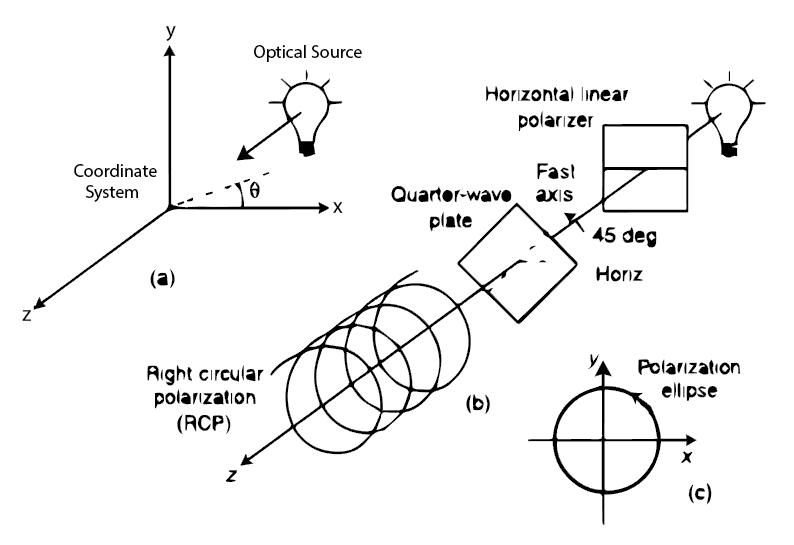Figure 2: A coordinate system for unambiguous description of the state of polarization

The z-axis is horizontally oriented in the direction of propagation of the light. The y-axis is vertically oriented pointing up, and the x-axis is horizontally oriented to form a right-handed rectangular coordinate system.

Angles are measured with reference to the x-axis, a positive angle indicating the sense of rotation from the x-axis toward the y-axis.

### The Polarization Ellipse

Here we consider a light beam of monochromatic (single wavelength) plane waves propagating in an isotropic and homogeneous medium. The beam can be represented by its electric field E(r,t), which can be writtenwhere ω is the angular frequency, k is the wave vector,  and A is a constant vector representing the amplitude.

The magnitude of the wave vector k is related to the frequency by the following equation:where n is the index of refraction of the medium, c is the speed of light in vacuum, and λ is the wavelength of light in vacuum. For materials with some absorption, the index of refraction is a complex number.

Reflecting the transverse nature, the electric field vector is always perpendicular to the direction of propagation,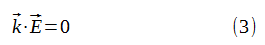For mathematical simplicity, the monochromatic plane wave in equation (1) is often written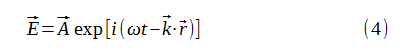with the understanding that:

only the real part of the right side represents the actual electric field. This is just an analytic representation.

The time evolution of the electric field vector is exactly sinusoidal; that is, the electric field must oscillate at a definite frequency.

For the purpose of describing various representations of the polarization states, we consider propagation along the z axis. Being a transverse wave, the electric field vector must lie in the xy plane. The two mutually independent components of the electric field vector can be written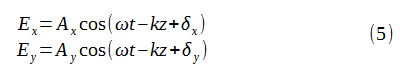where the two independent and positive amplitudes Ax and Ay, and the two independent phases δa and δy, reflect the mutual independence of the two components. With the amplitudes being positive, the phase angles are defined in the range -π < δx, δy ≤ π.

Since the x component and the y component of the electric field vector can oscillate independently at a definite frequency, one must consider the effect produced by the vector addition of these two oscillating orthogonal components.

The orbit of a general motion is an ellipse, which corresponds to oscillations in which the x and y components are not in phase. For optical waves, the corresponds to elliptic polarization states.

• Linear polarization states
• Circular polarization states

Without loss of generality, we consider the time evolution of the electric field vector at the origin z = 0. According to equation (5), the electric field components are writtenWe define a relative phase aswhere, again, δ is limited to the region  -π < δ ≤ π.

#### Linear Polarization States

A beam of light is said to be linearly polarized if the electric field vector vibrates in a constant direction (in the xy plane). This happens when the two components of oscillation are in phase (δ = δy - δx = 0), or out of phase by π (δ = δy - δx = π).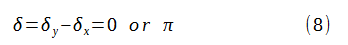In this case, the electric field vector vibrates sinusoidally along a constant direction in the xy plane defined by the ratio of the two components,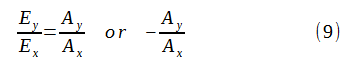Since the amplitudes Ax and Ay are independent, the electric field vector of linearly polarized light can vibrate along any direction in the xy plane.

#### Circular Polarization States

The other special case of importance is that of the circular polarization state. A beam of light is said to be circularly polarized if the electric field vector undergoes uniform rotation in the xy plane. This occurs when: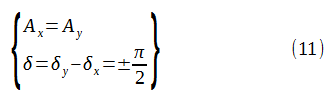δ = -π/2    ----> the light beam is right-handed circularly polarized (counterclockwise rotation)

δ = π/2     ----> the light beam is left-handed circularly polarized (clockwise rotation)Our convention for labeling right-handed and left-handed polarization is consistent with the terminology of modern physics in which a photon with a right-handed circulation polarization has a positive angular momentum along the direction of propagation.

It is interesting to note that the conditions of equal amplitude and +/- π/2 phase shift for circular polarization states are valid in any set of perpendicular coordinates in the xy plane. This means that when the electric field vector of a circularly polarized light is decomposed into any two mutually perpendicular components, the amplitudes are always equal and the phase shift is always +/- π/2.

#### Elliptic Polarization States

Both linear and circular polarization states are actually special cases of elliptic polarization states.

A light beam is said to be elliptically polarized if the curve traced by the end point of the electric field vector is an ellipse (in the xy plane) as shown below.

At a given point in space (say, z = 0), equation (5) is a parametric representation of an ellipse traced by the end point of the electric field vector. The equation of the ellipse can be obtained by eliminating ωt in equation (6).Equation (12) is an equation of a conic. From equation (6) we can see that this conic section is confined in a rectangular region with sides parallel to the coordinate axes and whose lengths are 2Ax and 2Ay. Therefore, the curve is an ellipse.

Thus, we find that the polarization states of light are, in general, elliptical.

A complete description of an elliptical polarization state includes the orientation of the ellipse relative to the coordinate axes and the shape and sense of revolution of the electric field vector.

In general, the principle axes of the ellipse are not in the x and y directions. By using a transformation (rotation) of the coordinate system, we are able to diagonalize equation (12).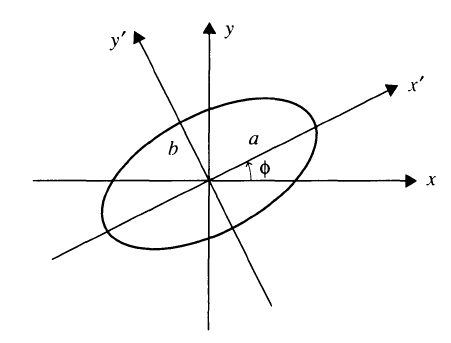Figure 3: Polarization Ellipse

Let x' and y' be the new set of  axes along the principal axes of the ellipse. Then the equation of the ellipse in this new coordinate system becomes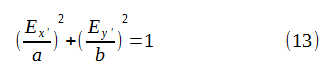where a and b are the lengths of the principal semiaxes of the ellipse, and Ex' and Ey' are the components of the electric field vector in this principal coordinate system.

Let Φ be the angle between the x' axis and the x axis. Then the lengths of the principal axes are given by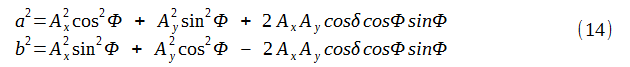The angle Φ can be expressed in terms of Ax, Ay, and cosδ asIt is important to note that Φ+π/2 is also a solution, if Φ is a solution of the equation.

Revolution Direction of an Elliptical Polarization

The revolution direction of an elliptical polarization is determined by the sign of sinδ. The end point of the electric field vector will revolve in a clockwise direction if  sinδ > 0, and in a counterclockwise direction if sinδ < 0.

The following figure shows how the polarization ellipse changes with varying phase difference δ.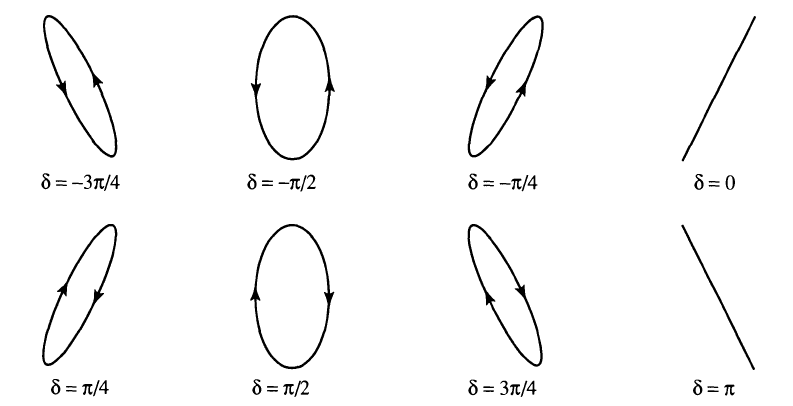Figure 4: Polarization ellipses at various phase angles δ

Ex = 1/2 cos(ωt - kz)
Ey = cos(ωt - kz + δ)

Ellipticity of an Elliptical Polarization

The ellipticity of a polarization ellipse is defined aswhere a and b are the lengths of the principal semiaxes. The ellipticity is taken as positive when the rotation of the electric field vector is right handed, and negative if left handed. For circularly polarized light, e = +/-1.

An elliptic polarization state can always be decomposed into two mutually orthogonal components. The relative phase shift between these two components can be anywhere between -π and π.

However, in the principal coordinate system (x', y' system after rotation), the relative phase shift between the two orthogonal components is always -π/2 or π/2 (depending on the revolution direction).